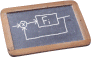# Introduction to Machine Learning

COURSE DESCRIPTION

Objectives

The goal of the course is to provide a concise introduction to the most popular and practical techniques for learning mathematical models from data. Different methods for solving function regression, classification, and clustering problems will be illustrated, understanding their mathematical foundations, the underlying learning algorithms, and how to validate their prediction performance. Examples from different application domains and how to solve them in Python will be shown during the course to illustrate the concepts.

Syllabus

Introduction to machine learning: supervised/unsupervised learning, classification/regression, overfitting, bias/variance tradeoff, cross-validation, examples. Linear regression and least squares: loss functions and regularization, basis functions and Kernel least squares, support vector regression, recursive least squares. Linear and Bayesian classification: ridge classifier, logistic regression, support vector classification, naive Bayes classifier. Non-parametric regression and classification: nearest neighbors, decision trees, Gaussian process regression, ensemble methods (bagging, bootstrap, random forests and feature importance, boosting methods). Neural networks: feedforward networks, backpropagation and automatic differentiation, learning algorithms (stochastic gradient descent, nonlinear least squares), AutoML; temporal convolutional networks, recurrent neural networks. Unsupervised learning: clustering methods (K-means clustering, density-based spatial clustering), dimensionality reduction (principal component analysis, nonlinear PCA), autoencoders.

PREREQUISITES

Basics of calculus, linear algebra, numerical optimization, probability theory, computer programming.

TIMETABLE
TBA
LOCATION

TBA

LECTURE SLIDES
• Introduction to machine learning
• Linear models for regression
• Classification: linear models, naïve Bayes
• Nonparametric models for classification and regression
• Neural networks
• Unsupervised learning (clustering, dimensionality reduction)
Last update: September 11, 2021